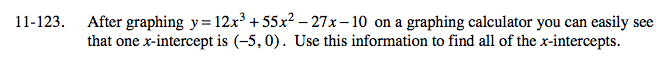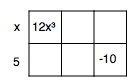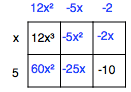Home > A2C > Chapter 11 > Lesson 11.2.6 > Problem11-123

11-123.

After graphing y = 12x3 + 55x2 − 27x − 10 on a graphing calculator you can easily see that one x-intercept is (−5, 0). Use this information to find all of the x-intercepts. Homework Help ✎This means that (x + 5) is a root, so you can divide:

$(-5,0\,)\left(\frac{2}{3},\,0\right)\left(-\frac{1}{4},\,0\right)$